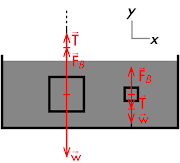## 20151121

### Physics quiz question: hanging vs. anchored submerged objects

Physics 205A Quiz 5, fall semester 2015
Cuesta College, San Luis Obispo, CATwo objects of different volumes and different densities are in a tank of water. One object hangs down from a string, while the other object is anchored to the bottom with a string. The amount of tension in each string is the same. The __________ object has a greater magnitude buoyant force exerted on it.
(A) hanging.
(B) anchored.
(C) (Not enough information is given.)
(D) (There is a tie.)

Correct answer (highlight to unhide): (A)

The magnitude of the buoyant force is given by:

FB = ρfluid·g·(Volume submerged),

where the density ρ is of the surrounding fluid (water) and the gravitational constant g = 9.80 m/s2. Both objects are fully submerged, and since the volume of water displaced by the hanging object is greater than the volume of water displaced by the anchored object, then the hanging object has a greater magnitude buoyant force exerted on it.

(The list of the forces acting on the hanging object are:
Buoyant force of water FB = ρfluid·g·(Volume submerged) (upwards),
Tension force of string T (upwards),
Weight force of Earth w = m·g (downwards).
The list of the forces acting on the hanging object are:
Buoyant force of water FB = ρfluid·g·(Volume submerged) (upwards),
Tension force of string T (downwards),
Weight force of Earth w = m·g (downwards).Newton's first law applies to both objects, as they are stationary, and thus the forces acting on either object must sum to zero.

For the hanging object, the magnitude of the downward weight force w must equal the sum of the magnitudes of the upwards tension force T and (larger) upwards buoyant force FB, and thus the hanging object will have a larger magnitude weight force than the anchored object (which has a downward weight force w equal to the (smaller) upwards buoyant force FB minus the tension force T.)

Sections 70854, 70855, 73320
Exam code: quiz05bL8D
(A) : 18 students
(B) : 29 students
(C) : 17 students
(D) : 1 student

Success level: 27%
Discrimination index (Aubrecht & Aubrecht, 1983): 0.80